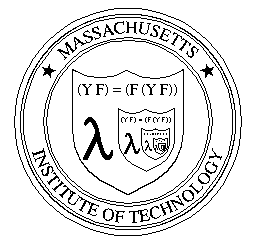# 康托尔、哥德尔、图灵——永恒的金色对角线(rev#2)

By 刘未鹏
C++ 的罗浮宫

—— 康托尔

—— 无名氏

bool God_algo(char* program, char* input)
{
if(<program> halts on <input>)
return true;
return false;
}

bool Satan_algo(char* program)
{
if( God_algo(program, program) ){
while(1); // loop forever!
return false; // can never get here!
}
else
return true;
}

Satan_algo(Satan_algo);

Satan_algo(Satan_algo) 能够停机 => 它不能停机
Satan_algo(Satan_algo) 不能停机 => 它能够停机

Y Combinatorlambda calculus

<expr> ::= <identifier>
<expr> ::= lambda <identifier-list>. <expr>
<expr> ::= (<expr> <expr>)

lambda x y. x + y

haskell 里面为了简洁起见用 “/” 来代替希腊字母 lambda ，它们形状比较相似。故而上面的定义也可以写成：
/ x y. x + y

((lambda x y. x + y) 2 3)

let Add = (lambda x y. x + y)

Alpha 转换公理 ：例如， “lambda x y. x + y” 转换为 “lambda a b. a + b” 。换句话说，函数的参数起什么名字没有关系，可以随意替换，只要函数体里面对参数的使用的地方也同时注意相应替换掉就是了。
Beta 转换公理 ：例如 “(lambda x y. x + y) 2 3” 转换为 “2 + 3” 。这个就更简单了，也就是说，当把一个 lambda 函数用到参数身上时，只需用实际的参数来替换掉其函数体中的相应变量即可。

f(n):
if n == 0 return 1
return n*f(n-1)

lambda n. If_Else n==0 1 n*<self>(n-1)

let F = lambda n. IF_Else n==0 1 n*F(n-1)

lambda n. If_Else n==0 1 n*<self>(n-1)

lambda self n. If_Else n==0 1 n*self(n-1)

let P = lambda self n. If_Else n==0 1 n*self(n-1)

P (P, 3)

IF_Else n==0 1 n*P(n-1)

let P = lambda self n. If_Else n==0 1 n*self(self, n-1)

IF_Else 3==0 1 3*P(P, 3-1)

P (P, 3-1) 是对 P 合法的递归调用。这次我们真的成功了！

let P = lambda self n. If_Else n==0 1 n*self(n-1)

power(n):
if(n==0) return 1;
return n*power(n-1);

P(power, 3)

IF_Else 3==0 1 3*power(3-1)

P(power)

IF_Else n==0 1 n*power(n-1)

IF_Else n==0 1 n*power(n-1)

lambda n. IF_Else n==0 1 n*power(n-1)

let power = lambda n. IF_Else n==0 1 n*power(n-1)

P(power) = power

let P = lambda self n. If_Else n==0 1 n*self(n-1) // 注意， 不是 self(self,n-1)

Y(F) = f

Y(F) = f = F(f) = F(Y(F))

Y(F) = F(Y(F))

let P = lambda self n. If_Else n==0 1 n*self(n-1)

power = P(power) // 不动点原理

let power_gen = lambda self. P(self(self))

power_gen(power_gen)

P (power_gen(power_gen))

power_gen(power_gen) => P(power_gen(power_gen))

power => P(power)

OK ，我们总结一下：对于给定的 P ，只要构造出一个相应的 power_gen 如下：

let power_gen = lambda self. P(self(self))

let Y = lambda F.
let f_gen = lambda self. F(self(self))
return f_gen(f_gen)

let Pwr = lambda self n. If_Else n==0 1 n*self(n-1)

Y(Pwr) =>
let f_gen = lambda self. Pwr(self(self))
return f_gen(f_gen)

Y(Pwr) 的求值结果就是里面返回的那个 f_gen(f_gen) ，我们再根据 f_gen 的定义展开 f_gen(f_gen) ，得到：

Pwr(f_gen(f_gen))

Y(Pwr) => f_gen(f_gen) => Pwr(f_gen(f_gen))

Pwr(f_gen(f_gen)) => If_Else n==0 1 n*f_gen(f_gen) (n-1)

Pwr(f_gen(f_gen)) => If_Else n==0 1 n* Pwr(f_gen(f_gen)) (n-1)

f => If_Else n==0 1 n*f(n-1)

N(n) is unprovable in T

UnPr( N(n) )

G(n): UnPr( N(n) )

G(g): UnPr( G(g) )

G(n): UnPr( N(n) )

G(n): P( N(n) )

G(g): P( G(g) )

1 2 3 4 …
2 4 6 8 …

1/1  1/2  2/1  1/3  2/2  3/1  1/4  2/3 3/2 4/1 …

1  a 10 .a11a12a13
2  a 20 .a21a22a23
3  a 30 .a31a32a33
4 …
5 …
（注： aij 里面的 ij 是下标

1    2     3    4   …
M1  N    1    N    N   …
M2  2    0     N    0   …
M3  0    1     2    0    …
M4  N    0     5    N   …

M1 M2 M3 … 是逐一列出的图灵机，并且，注意，由于程序即数据，每个图灵机都有唯一编码，所以我们规定在枚举图灵机的时候 Mi 其实就代表编码为 i 的图灵机，当然这里很多图灵机将会是根本没用的玩意，但这不要紧。此外，最上面的一行 1 2 3 4 … 是输入数据，如，矩阵的第一行代表 M1 分别在 1 2 3 上面的输出，不停机的话就是 N

P(i):
if( H(i, i) == 1 ) then // Mi(i) halts
return 1 + Mi(i)
else // if H(i, i) == 0 (Mi(i) doesn’t halt)
return 0

Mk(k) = P(k) =
1+Mk(k) if Mk(k) halts
0       if Mk(k) doesn’t halt

R = {X:X 不属于 X};

S1  S2  S3 …
S1 0   1  1 …
S2 1   1   0 …
S3 0   0   0 …
…    …

P.S
1 . lambda calculus 里面的 停机问题

2 . 负喧琐话 ( 是个非常不错的 blog:) g9 的文字轻松幽默，而且有很多名人八卦可以养眼，真的灰常 灰常 不错哦。此外 g9 老兄还是个理论功底非常扎实的牛。所以， anyway ，看了他的 blog 就知道啦！最初这篇文章的动机也正是看了上面的一篇 关于Y Combinator的铸造过程的介绍 ，于是想揭示一些更深的东西，于是便有了本文。
3. 文章起名《康托尔、哥德尔、图灵 —— 永恒的金色对角线》其实是为了纪念看过的一本好书 GEB ，即《 Godel Escher Bach-An Eternal Golden Braid 》中文译名《哥德尔、埃舍尔、巴赫 —— 集异璧之大成》 —— 商务印书馆出版。对于一本定价 50 元居然能够在 douban 上卖到 100 元的二手旧书，我想无需多说。另，幸福的是，电子版可以找到 :)
4 . 其实很久前想写的是一篇《从哥德尔到图灵》，但那篇写到 1/3 不到就搁下了，一是由于事务，二是总觉得少点什么。呵呵，如今把康托尔扯进来，也算是完成当时扔掉的那一篇吧。
5. 这恐怕算是写得最曲折的一篇文章了。不仅自己被这些问题搞得有点晕头转向（还好总算走出来），更因为要把这些东西自然而然的串起来，也颇费周章。很多时候是利用吃饭睡觉前或走路的时间思考本质的问题以及如何表达等等，然后到纸上一气呵成。不过同时也锻炼了不拿纸笔思考数学的能力，呵呵。
6. 关于图灵的停机问题、 Y Combinator 、哥德尔的不完备性定理以及其它种种与康托尔的对角线之间的本质联系，几乎查不到完整系统的深入介绍，一些书甚至如《 The Emperor’s New Mind 》也只是介绍了与图灵停机问题之间的联系（已经非常的难得了）， google baidu 的结果也是基本没有头绪。很多地方都是一带而过让人干着急。所以看到很多地方介绍这些定理和构造的时候都是弄得人晕头转向的，绝大部分人在面对如 Y Combinator 、不完备性定理、停机问题的时候都把注意力放在力图理解它是怎么运作的上面了，却使人看不到其本质上从何而来，于是人们便对这些东东大为惊叹。这使我感到很不痛快，如隔靴搔痒般。这也是写这篇文章的主要动机之一。

Reference
 《数学——确定性的丧失》
 也有观点认为函数式编程语言之所以没有广泛流行起来是因为一些实际的商业因素。
 Douglas R.Hofstadter的著作《Godel, Escher, Bach: An Eternal Golden Braid》（《哥德尔、艾舍尔、巴赫——集异璧之大成》）就是围绕这一思想写出的一本奇书。非常建议一读。
 《数学——确定性的丧失》
 虽然我觉得那个系徽做得太复杂，要表达这一简洁优美的思想其实还能有更好的方式。
 关于如何在lambda calculus系统里实现“+”操作符以及自然数等等，可参见 这里， 这里，和 这里。
 g9的blog（负暄琐话） http://blog.csdn.net/g9yuayon/ 上有一系列介绍lambda calculus的文章（当然，还有其它好文章:)），非常不错，强烈推荐。最近的两篇就是介绍Y combinator的。其中有一篇以javaScript语言描述了迭代式逐步抽象出Y Combinator的过程。
 实际上这只是第一不完备性定理，它还有一个推论，被称为第二不完备性定理，说的是任一个系统T内无法证明这个系统本身的一致性。这个定理的证明核心思想如下：我们前面证明第一不完备性定理的时候用的推断其实就表明 Con/T -> G(g) （自然语言描述就是，由系统T的无矛盾，可以推出G(g)成立），而这个“Con/T -> G(g)”本身又是可以在T内表达且证明出来的（具体怎么表达就不再多说了）——只需要用排中律即可。于是我们立即得到，T里面无法推出Con/T，因为一旦推出Con/T就立即推出G(g)从而推出UnPr(G(g))，这就矛盾了。所以，Con/T无法在T内推出（证明）。

08-05528
09-03309
12-2536
12-17343
01-12160
05-202127
08-14294
08-07792
10-217574
11-22248
11-181287
02-18296
04-1942
02-26648
10-161418
02-18272

### “相关推荐”对你有帮助么？

•非常没帮助
•没帮助
•一般
•有帮助
•非常有帮助pongba

¥2 ¥4 ¥6 ¥10 ¥20余额支付 (余额：-- )扫码支付获取中扫码支付点击重新获取扫码支付1.余额是钱包充值的虚拟货币，按照1:1的比例进行支付金额的抵扣。
2.余额无法直接购买下载，可以购买VIP、C币套餐、付费专栏及课程。余额充值